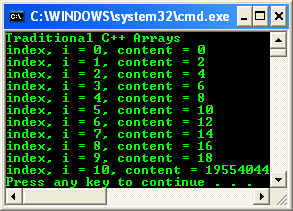2. # Native C++ Arrays

---------Next------------------------

19. # Array.BinarySearch Method (Array, Object) Example

Introduction

This module is concerned with data structures. You’ll learn about arrays and other collection classes, and you’ll learn how to use them in your programs. In the first part of the module, you’re going to learn about two sorts of arrays: the native arrays provided by the C++ language and the .NET managed arrays, which use functionality inherited from the .NET Framework. You’ll find out how they all work and when one should be used rather than the other. The second part of the module looks more widely at the range of collection classes provided by the .NET Framework, discussing their characteristics and showing you how and when to use them.

Native arrays are those provided as part of the C++ language. They’re based on the arrays that C++ inherits from C. Although native arrays are designed to be fast and efficient, there are drawbacks associated with using them, as you’ll see shortly. This first exercise will introduce you to C++ native arrays by showing you how to create an array of value types and how to use the array.

1.        Open Microsoft Visual C++/Studio .NET, and create a new CLR Console Application project named Trad.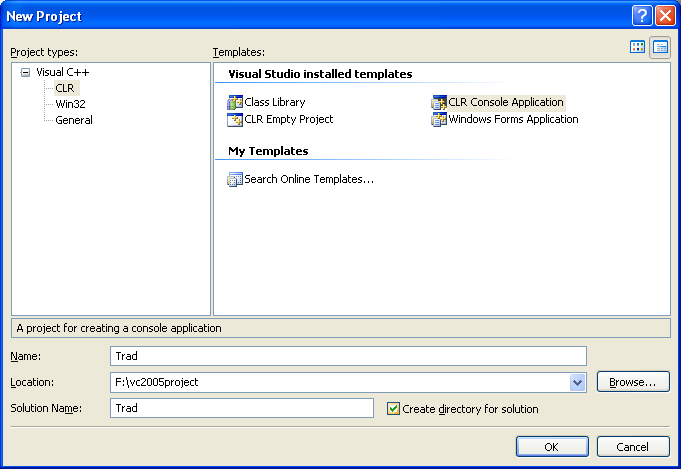2.        Open the source file Trad.cpp and add the following code to the main() function:

int main(array<System::String ^> ^args)

{

// Create an array

int arr;

// Fill the array

for(int i=0; i<10; i++)

arr[i] = i*2;

return 0;

}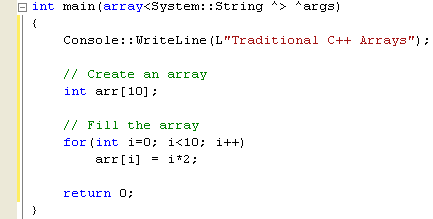The array is created by giving a type, a name, and a size enclosed in square brackets ([]). Here the array is named arr and it holds ten int values. All arrays are created using the same syntax, as shown here:

// Create an array of six doubles

double arr;

// Create an array of two char*’s

char* arr;

Here’s the first important point about native arrays: once you’ve created an array, you can’t resize it, so you have to know how many elements you’re going to need before you start. If you don’t know how many elements you’re going to need, you might be better off using a .NET array, discussed later in this module.

The array size has to be known at compile time, so, for example, you can’t ask the user for a value and then use that value to specify an array dimension at run time. However, it’s common to create constants, either by using preprocessor #define declarations or by declaring const int variables, and using them to specify array dimensions. For example:

#define size 100

As you can see from the loop in the preceding code, array elements are accessed using square brackets that contain the index. Here’s the second important point about native arrays: indexing starts from zero rather than one, so the valid range of indices for an array is from zero to one less than the size of the array. In other words, for a 10-element array, valid indices are  to .

3.        Add a second loop to print out the array’s contents after filling it.

 // Print its contents for(int j=0; j<10; j++)    Console::WriteLine(arr[j]);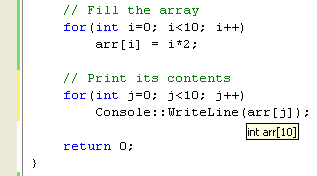4.        Build and run your program. You should find that the values print, one to a line, as shown in the following figure.What happens if you change the range of the second loop so that it tries to print the element at ?

5.        Alter the code in the second loop to look like this:

// Print its contents

for(int j=0; j<=10; j++)

Console::WriteLine(arr[j]);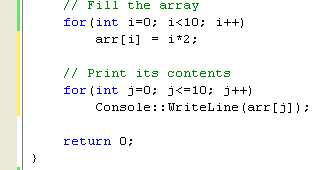Notice the less than or equal to (<=) condition. The effect of this condition is to try to print 11 elements rather than 10.

6.        Compile and run the program, and you should see output similar to the following figure.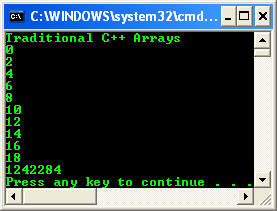Notice the random value that’s been printed at the end of the list. Here’s the third important point about native arrays: bounds aren’t checked. Native arrays in C++ aren’t objects and therefore they have no knowledge of how many elements they contain. It’s up to you to keep within the bounds of the array, and if you don’t, you risk corrupting data, generating buffer overflow and crashing your program. As a security and normal practice, programmers will provide codes that check the limit.

7.        To make the previous code ‘cleaner’ and meaningful, the following is the modified version.

// Print its contents

for(int j=0; j<=10; j++)

Console::WriteLine("index, i = {0}, content = {1}", j, arr[j]);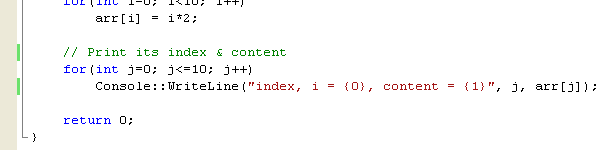The output is shown below.